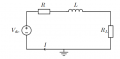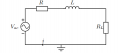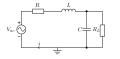# Calculation of power factor in a RLC-circuit

#### cooltafel

Joined Mar 20, 2018
8
Hello,

I got a homework exercise about the calculation of the power factor in a RL-circuit and in a RLC-circuit.
The question consists of 3 sub questions, I have managed to calculate the first 2 however I am struggling with the third one.
For the calculation of the third question, the other 2 questions are also needed.

Question 1
In the circuit below the given values are:
V_dc = 24V, R = 6.37 ohm, L = 115,76 mH, R_L = 30 ohm
Assume that all circuit elements are ideal components.The question is to calculate the impedanze Z_eq, as seen by the source, and the current I.
Solution:
Since there is a DC voltage, the inductor L behaves as a wire. The impedance of L is X_L = 2*pi*0*115,76*10^-3 = 0, because of the DC voltage.
Thus Z_eq = 6.37 + 30 = 36.37 ohm
The current I is then I = 24/36.37 A = 0.65988 A

Question 2
The DC voltage source is replaced by an AC voltage source, that generates a sinusoidal vol andtage has a frequency of 50 Hz and a peak voltage of 325V.
The question is to calculate the impedance Z_eq, the current i and the power factor.Solution
First of all, calculating X_L. X_L = 2*pi*50*115,76*10^-3 ohm
Z_eq = sqrt((X_L)^2+(R+R_L)^2) giving Z_eq = 51.43288 ohm

Then calculating the V_rms:
V_rms = V_peak / sqrt(2) = 325/sqrt(2)
i = 325/sqrt(2) / Z_eq = 4.46815 A

Eventually the power factor:
P = I^2*R = (4.46815)^2*36.37 = 726.10393 W
S = V^2/Z = 1026.82370 W
Power factor: P_f = P/S = 726.10393/1026.82370 = 0.70714

Question 3
Now to improve the power factor, a capacitor is added to the circuit.
C = 52 µF
The question is to calculate the improves power factor.Solution
I think the steps to take are:
1) Calculate impedance of C
2) Calculate the total impedance of C and R_L (parallel)
3) Calculate the total impedance of the circuit by summing the answer of step (2) and the answer of X_L + R
4) Since the AC voltage did not change, the V_rms can be reused from question 2 and the current can be calculated with the answer of step (3).
5) With all the calculated information the equation P_f = P/S can be calculated.

I don't know if this is the right way to calculate it, because I think at step 3 a difficult answer will be derived with fractions. I know that the power factor has improved by adding the capacitor C.

Please help me with the calculation. I don't want fully written out answers, but rather something that pushes me in the right way.

Thanks for the effort and time in advance!

#### MrAl

Joined Jun 17, 2014
9,174
Hello,

I got a homework exercise about the calculation of the power factor in a RL-circuit and in a RLC-circuit.
The question consists of 3 sub questions, I have managed to calculate the first 2 however I am struggling with the third one.
For the calculation of the third question, the other 2 questions are also needed.

Question 1
In the circuit below the given values are:
V_dc = 24V, R = 6.37 ohm, L = 115,76 mH, R_L = 30 ohm
Assume that all circuit elements are ideal components.
View attachment 191211
The question is to calculate the impedanze Z_eq, as seen by the source, and the current I.
Solution:
Since there is a DC voltage, the inductor L behaves as a wire. The impedance of L is X_L = 2*pi*0*115,76*10^-3 = 0, because of the DC voltage.
Thus Z_eq = 6.37 + 30 = 36.37 ohm
The current I is then I = 24/36.37 A = 0.65988 A

Question 2
The DC voltage source is replaced by an AC voltage source, that generates a sinusoidal vol andtage has a frequency of 50 Hz and a peak voltage of 325V.
The question is to calculate the impedance Z_eq, the current i and the power factor.
View attachment 191213
Solution
First of all, calculating X_L. X_L = 2*pi*50*115,76*10^-3 ohm
Z_eq = sqrt((X_L)^2+(R+R_L)^2) giving Z_eq = 51.43288 ohm

Then calculating the V_rms:
V_rms = V_peak / sqrt(2) = 325/sqrt(2)
i = 325/sqrt(2) / Z_eq = 4.46815 A

Eventually the power factor:
P = I^2*R = (4.46815)^2*36.37 = 726.10393 W
S = V^2/Z = 1026.82370 W
Power factor: P_f = P/S = 726.10393/1026.82370 = 0.70714

Question 3
Now to improve the power factor, a capacitor is added to the circuit.
C = 52 µF
The question is to calculate the improves power factor.
View attachment 191215

Solution
I think the steps to take are:
1) Calculate impedance of C
2) Calculate the total impedance of C and R_L (parallel)
3) Calculate the total impedance of the circuit by summing the answer of step (2) and the answer of X_L + R
4) Since the AC voltage did not change, the V_rms can be reused from question 2 and the current can be calculated with the answer of step (3).
5) With all the calculated information the equation P_f = P/S can be calculated.

I don't know if this is the right way to calculate it, because I think at step 3 a difficult answer will be derived with fractions. I know that the power factor has improved by adding the capacitor C.

Please help me with the calculation. I don't want fully written out answers, but rather something that pushes me in the right way.

Thanks for the effort and time in advance!
Hello,

The simpler way is to just calculate the phase angle TH then the power factor is:
PF=cos(TH)

where the phase angle TH is:
TH=atan2(imagpart,realpart)

where imagpart and realpart are the imaginary part and real part of the impedance and
atan2(i,r) is the two argument inverse tangent function.

If you want to do it the other way then:
PF=realpower/apparentpower

where realpower is in watts and apparentpower is in volt amps so it is W/VA.

For this particular problem you should get a new PF of between 0.75 and 0.85 but i wont give the exact number out yet until you try this.

Last edited:

#### Jony130

Joined Feb 17, 2009
5,351
For this particular problem you should get a new PF of less than 0.001
Are you sure? Because I've got a different value.

#### MrAl

Joined Jun 17, 2014
9,174
Are you sure? Because I've got a different value.
Hi,

Ok i'll check again just in case.
I am calculating the complete power factor as seen by the source.

LATER:
Ok some how i used the wrong value for L.
Now i get between 0.70 and 0.80 i wont say exactly just yet.
Previous post corrected.

I also get optimum C a bit less than 52uf.

Last edited:

#### cooltafel

Joined Mar 20, 2018
8
Hey,

First of all, thank you everyone for your replies! I appreciate it.

I have tried to calculate the problem with the formula Pf = realpower/apparentpower

I think I might have made some errors somewhere, but here are the steps I made:

1) Calculate the complex impedance of the capacitor: X_c = -j/(2*pi*50*52*10^-6) = -61.21344j ohm
2) Then I added the parallel impedance (X_c) and the load resistor (R_L):
X_c || R_L = ((1/30) + (1/-61.21344j))^-1 ohm
It results in -39.48338j - 19.35035
3) Then I calculated the impedance of the inductor and resistor, which equals to: 6.37 + 0.0014739j
4) The total impedance is then equal to Z_eq = sqrt((39.48486)^2+(25.72035)^2) = 47.12314 ohm
5) Then the current i is 325/(sqrt(2)*47.12314) = 4.87679 A
6) Pf = P/S
P = i^2*(R||R_L) = (4.87679)^2*5.25433 = 124.9642 W
S = (325/sqrt(2))^2/47.12314 = 1120.733 VA

Thus Pf = 0.1115

This value does not correspond with the value MrAI says.

Perhaps the phase angle calculation gives me a better result, but I have to look into how it works.

#### Jony130

Joined Feb 17, 2009
5,351
2) Then I added the parallel impedance (X_c) and the load resistor (R_L):
X_c || R_L = ((1/30) + (1/-61.21344j))^-1 ohm
It results in -39.48338j - 19.35035
https://www.wolframalpha.com/input/?i=+((1/30)+++(1/-61.21344+i))^-1+

Then I calculated the impedance of the inductor and resistor, which equals to: 6.37 + 0.0014739j
What? Did you change the inductance value? Because the inductive reactance is equal to XL = 36.36Ω

The total impedance is then equal to Z_eq = sqrt((39.48486)^2+(25.72035)^2) = 47.12314 ohm
I'm not sure if this is allowed, it will be better if you continue your calculations in complex form and at the end, you take the magnitude of a complex number (Z_eq).

Last edited:

#### MrAl

Joined Jun 17, 2014
9,174
Hey,

First of all, thank you everyone for your replies! I appreciate it.

I have tried to calculate the problem with the formula Pf = realpower/apparentpower

I think I might have made some errors somewhere, but here are the steps I made:

1) Calculate the complex impedance of the capacitor: X_c = -j/(2*pi*50*52*10^-6) = -61.21344j ohm
2) Then I added the parallel impedance (X_c) and the load resistor (R_L):
X_c || R_L = ((1/30) + (1/-61.21344j))^-1 ohm
It results in -39.48338j - 19.35035
3) Then I calculated the impedance of the inductor and resistor, which equals to: 6.37 + 0.0014739j
4) The total impedance is then equal to Z_eq = sqrt((39.48486)^2+(25.72035)^2) = 47.12314 ohm
5) Then the current i is 325/(sqrt(2)*47.12314) = 4.87679 A
6) Pf = P/S
P = i^2*(R||R_L) = (4.87679)^2*5.25433 = 124.9642 W
S = (325/sqrt(2))^2/47.12314 = 1120.733 VA

Thus Pf = 0.1115

This value does not correspond with the value MrAI says.

Perhaps the phase angle calculation gives me a better result, but I have to look into how it works.

Well if you compare your PF before adding the capacitor and your PF now (0.1115) you can see that it got WORSE so i would think that would be wrong because it should have gotten better by at least a little.

The best power factor that can be obtained by placing a capacitor of the right value in that topological position is close to 0.7801 and so the actual power factor for this problem is very close to that value but again i refrain from giving the exact value until after you have had a chance to redo your calculations. Try again see what you can come up with this time. I got the same exact value doing it both ways.

#### cooltafel

Joined Mar 20, 2018
8
Hey,

Thank you again for your replies!

I have made big calculation errors. I did it on paper, but then realised I should use Matlab for the calculations.

The matlab code:

format long g

R_L = 30;
R = 6.37;
C = 52*10^-6;
L = 115.76 *10^-3;
V = 325/sqrt(2);
f = 50;

X_c = -j/(2*pi*f*C);
X_l = 2*pi*f*L*j;

Z_parallel = (1/X_c+1/R_L)^-1;
Z_eq = Z_parallel + X_l+R;

phi = atan2(imag(Z_eq),real(Z_eq))
Pf = cos(phi)

The code calculates the power factor by using the function atan2 which was proposed by MrAI.
This code gives the correct answer! I got 0.7801... now

Basically, I calculated the impedances of the capacitor and the inductor.
Then I calculated the impedance of the capacitor and load resistor which are in parallel.
With that I determined the Z_eq.
After that the function atan2(imag, real) did the magic for me.

Again thank you for your effort and time!

#### MrAl

Joined Jun 17, 2014
9,174
Hey,

Thank you again for your replies!

I have made big calculation errors. I did it on paper, but then realised I should use Matlab for the calculations.

The matlab code:

format long g

R_L = 30;
R = 6.37;
C = 52*10^-6;
L = 115.76 *10^-3;
V = 325/sqrt(2);
f = 50;

X_c = -j/(2*pi*f*C);
X_l = 2*pi*f*L*j;

Z_parallel = (1/X_c+1/R_L)^-1;
Z_eq = Z_parallel + X_l+R;

phi = atan2(imag(Z_eq),real(Z_eq))
Pf = cos(phi)

The code calculates the power factor by using the function atan2 which was proposed by MrAI.
This code gives the correct answer! I got 0.7801... now

Basically, I calculated the impedances of the capacitor and the inductor.
Then I calculated the impedance of the capacitor and load resistor which are in parallel.
With that I determined the Z_eq.
After that the function atan2(imag, real) did the magic for me.

Again thank you for your effort and time!
Hello again,

Actually i quoted that as being optimum for a capacitor value that is optimum, so the power factor for a cap of exactly 52uf would be slightly lower, very slightly. So could you post your result with a little more precision, like say 6 digits?
We could then compare.

Six digits with the optimum value capacitor is:
PF=0.780105

so the PF with C=52uf should be very slightly lower than that. See what you got.

#### cooltafel

Joined Mar 20, 2018
8
Hey MrAI,

The PF with C=52uf is:
0.780071

There indeed is a slight difference!

Is it possible to recreate the optimum capacitor with existing capacitor values ones?

Yet again thank you for the effort and time!

•Zeeus

#### MrAl

Joined Jun 17, 2014
9,174
Hey MrAI,

The PF with C=52uf is:
0.780071

There indeed is a slight difference!

Is it possible to recreate the optimum capacitor with existing capacitor values ones?

Yet again thank you for the effort and time!
Hello again,

Yes that's right now.

Do you mean create the optimum capacitor using capacitor values that are normally available on the market? I suppose that is possible. I dont they dont make a value that matches the optimum value though. Also, when we purchase capacitors there is a certain tolerance associated with each value anyway so in practice it probably would not work unless we experimented with different values until we got the optimum PF.

Here is what i get for the optimum C value to 13 digits:
C=5.091606935465e-5

so you see that exact value would be hard to get, and that is still only precise up to those 13 digits.
I would suppose you could do 5.09e-5 with some various cap values but since there are tolerances on the other parts too (R, RL, L) you would be better off measuring the PF and try different cap values, and then hopefully the values dont change that much with temperature.

Last edited:

#### rm_rocky

Joined May 24, 2015
1
Folks,
There's a red herring in this problem. The final circuit diagram as shown is impossible! The inductor has reactance and resistance but it is in one component. The capacitor cannot be wired across the reactance alone, it must be across both reactance and resistance. If you rework the problem with this different arrangement the power factor comes out very close to one. I believe this problem was designed to demonstrate the effectiveness of power factor compensation.